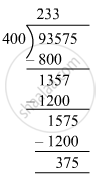# Divide, and find out the quotient and remainder. Check your answer. 93575 ​÷ 400 - Mathematics

Fill in the Blanks

93575 ​÷ 400

#### SolutionQuotient = 233 and Remainder = 375
Check: Divisor × Quotient + Remainder = 400 × 233 + 375
= 93575
= Dividend
∴ Dividend= Divisor × Quotient + Remainder

Concept: Concept for Whole Numbers
Is there an error in this question or solution?

#### APPEARS IN

RS Aggarwal Class 6 Mathematics
Chapter 3 Whole Numbers
Exercise 3E | Q 2.4 | Page 56